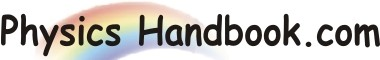HOME TOPICS DEFINITIONS TABLES LAWS INVENTIONS EXPERIMENTS QUIZ VIDEOS
 A B C D E F G H I J K L M N O P Q R S T U V W X Y Z
NOT Gate or Inverter
The NOT gate or Inverter is the simplest of all logic gates. It has only one input one output. Where the output Y opposite of the input. The NOT gate is often called inverter because it inverts the input.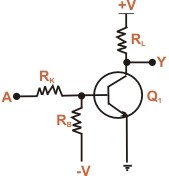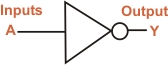Fig (a) Fig (b)
Fig (a) shows a typical inverter circuit. When A is connected to ground, the base of transistor Q1 will become negative. The negative potential cause the transistor to cut off and large positive voltage is applied at A, the base of the transistor will become positive, causing the transistor to conduct heavily. Therefore, the output voltage is zero. In binary terms, when A = 1,Y = 0. as shows truth table for an inverter. It is clear from the truth table that whatever the input to the inverter, the output assumes opposite polarity. If the input is 0, the output will be 1: if the input is 1,the output will be 0.
A
Y

0
1
1
0
Truth Table
Fig (b) shows the logic symbol for NOT gate or inverter. Note that small bubble on the inverter symbol represents inversion. The Boolean expression for NOT function is

Y =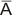Note that bar above the input A represents inversion.
If A = 0, then Y =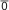or Y = 1
If A = 1, then Y =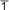or Y = 0